UIHistories Project: A History of the University of Illinois by Kalev Leetaru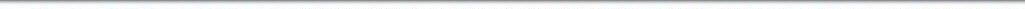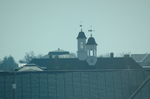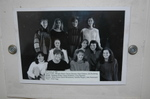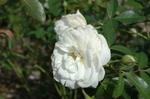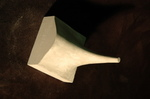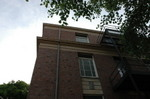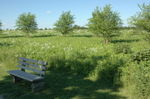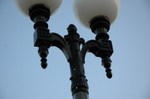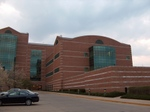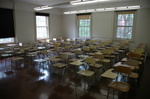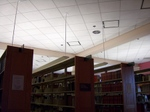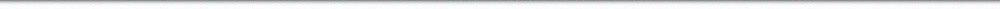N A V I G A T I O N D I G I T A L L I B R A R Y

## Repository: UIHistories Project: Board of Trustees Minutes - 1896 [PAGE 296]

Caption: Board of Trustees Minutes - 1896
This is a reduced-resolution page image for fast online browsing.

< Previous Page [Displaying Page 296 of 371] Next Page >
[VIEW ALL PAGE THUMBNAILS]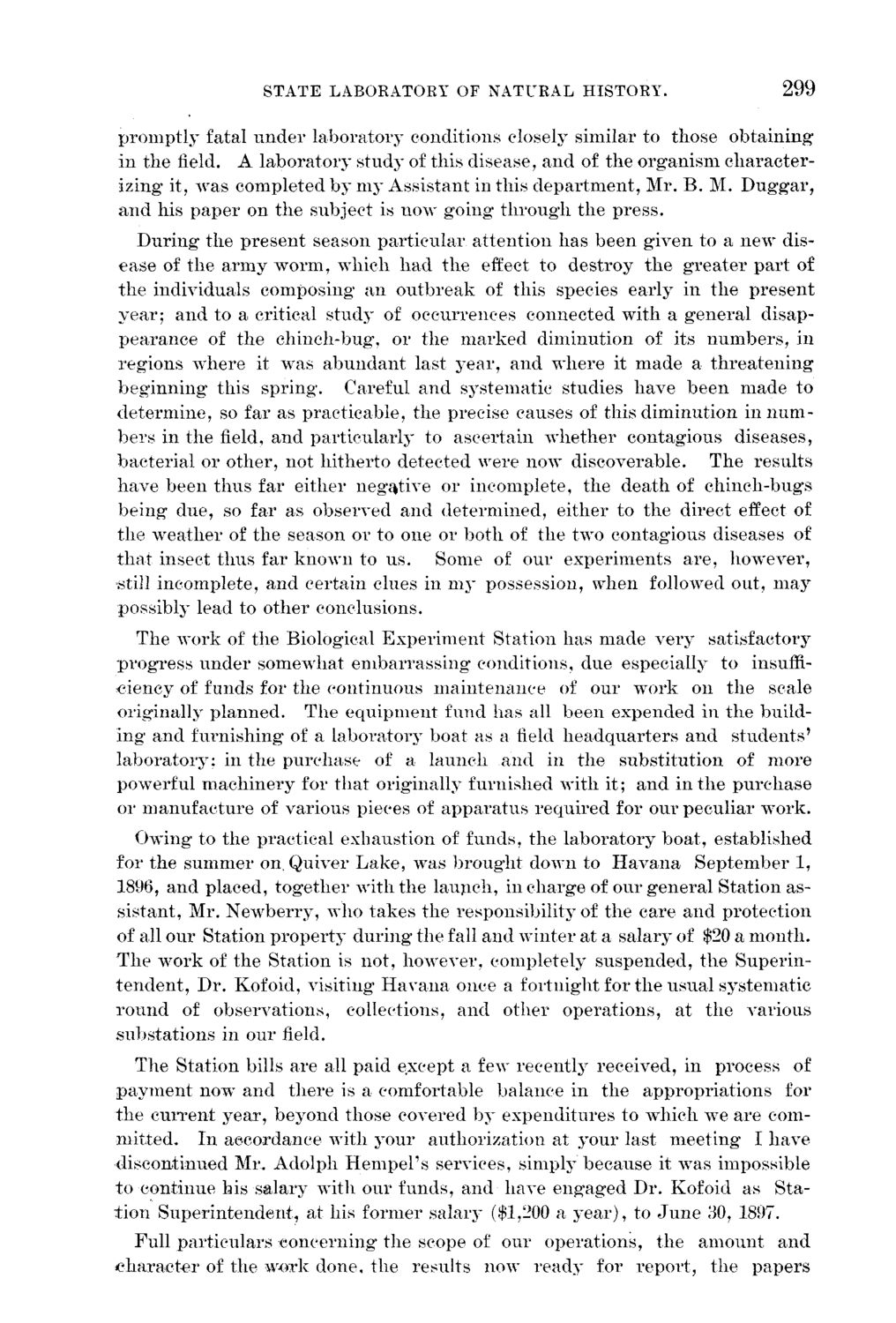### EXTRACTED TEXT FROM PAGE:

STATE LABORATORY OF NATURAL HISTORY.

299

p r o m p t l y fatal u n d e r laboratory conditions closely similar to those o b t a i n i n g in t h e field. A l a b o r a t o r y study of this disease, a n d of t h e o r g a n i s m c h a r a c t e r izing it, was completed b y m y Assistant in this d e p a r t m e n t , Mr. B . M. D u g g a r , a n d his p a p e r on t h e subject is now g o i n g t h r o u g h t h e p r e s s . D u r i n g the p r e s e n t season p a r t i c u l a r a t t e n t i o n h a s b e e n g i v e n to a n e w disease of t h e a r m y w o r m , which h a d t h e effect to d e s t r o y t h e g r e a t e r p a r t of t h e individuals composing a n o u t b r e a k of this species early in t h e p r e s e n t y e a r ; a n d to a critical study of occurrences connected with a g e n e r a l disapp e a r a n c e of t h e chinch-bug, or t h e m a r k e d diminution of its n u m b e r s , in r e g i o n s w h e r e it was a b u n d a n t last y e a r , a n d w h e r e it m a d e a t h r e a t e n i n g b e g i n n i n g this s p r i n g . Careful a n d systematic studies h a v e b e e n m a d e to d e t e r m i n e , so far as practicable, the precise causes of this diminution in n u m b e r s in the field, a n d p a r t i c u l a r l y to ascertain w h e t h e r contagious diseases, b a c t e r i a l or other, not hitherto detected were now discoverable. T h e results h a v e b e e n t h u s far either negative or incomplete, t h e d e a t h of c h i n c h - b u g s b e i n g d u e , so far as observed a n d d e t e r m i n e d , either to t h e direct effect of t h e w e a t h e r of t h e season or to one or b o t h of t h e two contagious diseases of t h a t insect t h u s far k n o w n to u s . Some of our e x p e r i m e n t s a r e , however, still incomplete, a n d certain clues in m y possession, when followed out, m a y possibly lead to other conclusions. T h e w o r k of t h e Biological E x p e r i m e n t Station h a s m a d e v e r y satisfactory p r o g r e s s u n d e r somewhat e m b a r r a s s i n g conditions, due especially to insuffic i e n c y of funds for t h e continuous m a i n t e n a n c e of our w o r k on t h e scale originally p l a n n e d . T h e e q u i p m e n t fund has all b e e n e x p e n d e d in t h e buildi n g a n d furnishing of a l a b o r a t o r y boat as a field h e a d q u a r t e r s a n d s t u d e n t s ' l a b o r a t o r y : in the p u r c h a s e of a l a u n c h a n d in t h e substitution of more powerful m a c h i n e r y for t h a t originally furnished w i t h it; a n d in t h e p u r c h a s e or m a n u f a c t u r e of various pieces of a p p a r a t u s r e q u i r e d for our peculiar w o r k . Owing to the practical exhaustion of funds, t h e l a b o r a t o r y b o a t , established for the s u m m e r on. Quiver L a k e , was b r o u g h t down to H a v a n a S e p t e m b e r 1, 1896, a n d placed, t o g e t h e r with t h e l a u n c h , in c h a r g e of our g e n e r a l Station assistant, Mr. N e w b e r r y , who t a k e s t h e responsibility of the care a n d protection of all our Station p r o p e r t y d u r i n g the fall a n d w i n t e r at a salary of \$20 a m o n t h . T h e w o r k of the Station is not, however, completely s u s p e n d e d , t h e S u p e r i n t e n d e n t , D r . Kofoid, visiting H a v a n a once a fortnight for t h e usual systematic r o u n d of observations, collections, a n d other o p e r a t i o n s , at t h e various s u b s t a t i o n s in our field. T h e Station bills are all paid except a few recently received, in process of p a y m e n t now a n d t h e r e is a comfortable balance in t h e a p p r o p r i a t i o n s for t h e c u r r e n t year, b e y o n d those covered b y e x p e n d i t u r e s to which we are comm i t t e d . I n accordance with y o u r authorization at y o u r last m e e t i n g I h a v e discontinued Mr. Adolpli H e m p e l ' s services, simply because it was impossible t o continue his salary with our funds, a n d have e n g a g e d Dr. Kofoid as Stat i o n S u p e r i n t e n d e n t , at his former salary (\$1,200 a y e a r ) , to J u n e 30, 1897. Full particulars c o n c e r n i n g the scope of our operations, t h e a m o u n t a n d c h a r a c t e r of the w o r k done, t h e results now r e a d y for r e p o r t , t h e p a p e r s